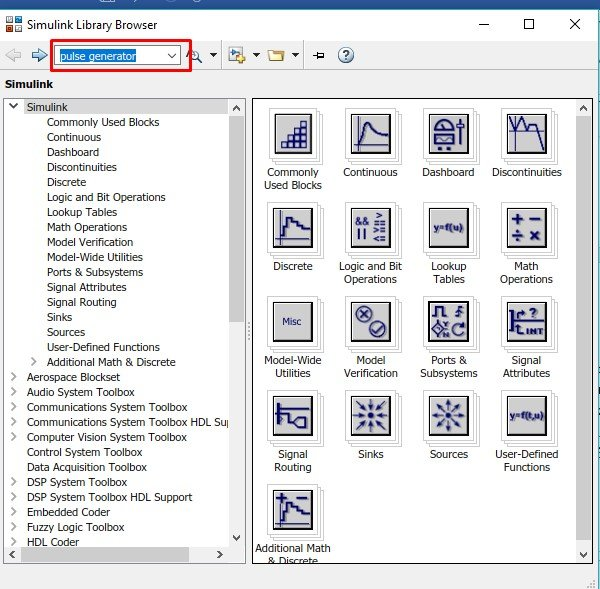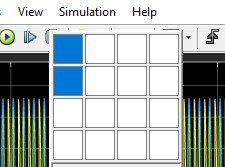# Implementation of controlled rectifiers in Simulink MATLAB

In this tutorial, I will explain you the working of controlled rectifiers and how we can implement them using MATLAB Simulink. Rectifiers are one of the very basic circuits of electronics however, controlled rectifiers are those whose output waveform can be controlled using electronic switches by controlling their firing angles. At the start a comprehensive introduction of controlled rectifiers is provided along with the types and uses of these. After that the circuit of controlled rectifiers is implemented on MATLAB’s Simulink. At the end you are provided with a simple exercise to perform it on your own regarding the concepts discussed in the tutorial.

## Introduction to Rectifiers

Controlled rectifiers are the basic components of power electronics. As the name suggests in a controlled rectifier we can control the shape of the output waveform of the rectifier with the help of switches. The waveform shape will depend on the firing angle of the switch connected to the bridge.

## Types of rectifiers

Controlled rectifiers can be classified into two major types

• Half wave rectifier
• Full wave rectifier.

### Half wave rectifier

Unlike simple half wave rectifier, half wave and full wave-controlled rectifiers depends on the number of switches used in the circuit. The pulses given to the switches have a specified pwm and that pwm will decide the shape of the rectified output wave, and the pwn of the pulse is also used to define the firing angle of the rectifier as you will see shortly.

### Full wave rectifier

A full wave rectifier however uses both the positive and negative parts of the AC wave to rectify. Again in this case we use switches with gate pulses to be used as the firing angles of the rectified output. The rectified output will not be a pure sinusoidal waveform although it will be a distorted sine 0 output before sinusoidal and rectified sine wave after firing angle of the pulses in the gate input of switches.

## controlled rectifiers in Simulink MATLAB

In the model, I am going to discuss with you we are going to design a Half wave controlled rectifier and the designing of full wave controlled rectifier is left as an exercise for you.

Open MATLAB to create a model and then open simulink by clinking on the icon present in the main page of MATLAB or by typing simulink in the command window of MATLAB. Once the simulink window pops up , click on the block named as blank model as we have been doing in the previous tutorials. After that open the library browser and in the search bar of library browser search “Pulse generator” as shown in the figure below, and press enter.Figure 1: Searching block

Figure 2: Pulse generator

Figure 3: Adding block to model

Figure 4: Power system

Figure 5: Specialized Technology

Figure 6: Fundamental blocks

Figure 7: Sources

Figure 8: AC voltage source

Figure 9: Power electronics

Figure 10: Thyristors

Figure 11: Power gui

Figure 12: Measurement block

Figure 13: Voltage Measurement

Figure 14: Elements block

Figure 15: Series RLC branch

Figure 16: Scope

Figure 17: Place component

Figure 18: Bridge

Figure 19: Pulse generator connected

Figure 20: No. of input ports

Figure 21: Complete block diagram

Figure 22: Parameters of pulse generator

Figure 23: Source block parameters

Figure 24: Changing layoutFigure 25: Selecting 2 blocks for layout

Figure 26: Output

• You can change the angle of control by changing the phase delay in pulse generator parameter.

Exercise:

• Perform the analysis on full wave controlled rectifier using MATLAB’s Simulink. It would be much simpler than the one performed in this tutorial.

(Hint: Number of diodes to be used will be two)

### 1 thought on “Implementation of controlled rectifiers in Simulink MATLAB”

1. How can view output vtg value